Two identical thin bar magnets each of length l and pole strength m are placed at the right angle to each other with the north pole of one touching south pole of the other. The magnetic moment of the system is :

1. ml

2. 2ml

3. $\sqrt{2}ml$

4. $\frac{1}{2}ml$

Concept Questions :-

Bar magnet
High Yielding Test Series + Question Bank - NEET 2020

Difficulty Level:

Rate of change of torque  with deflections maximum for a magnet suspended freely in a uniform magnetic field of induction B, when

(a) $\theta ={0}^{o}$                        (b) $\theta ={45}^{o}$

(c) $\theta ={60}^{o}$                      (d) $\theta ={90}^{o}$

Concept Questions :-

Bar magnet
High Yielding Test Series + Question Bank - NEET 2020

Difficulty Level:

Force between two identical bar magnets whose centres are r metre apart  is 4.8 N, when their axes are in the same line. If separation is increased to 2r, the force between them is reduced to

1. 2.4N                         2. 1.2N

3. 0.6N                         4. 0.3N

Concept Questions :-

Analogy between electrostatics and magnetostatics
High Yielding Test Series + Question Bank - NEET 2020

Difficulty Level:

A bar magnet of magnetic moment 104J/T is free to rotate in a horizontal plane. The work done in rotating the magnet slowly from a direction parallel to a horizontal magnetic field of 4×10–5 T to a direction 60° from the field will be

1. 0.2 J                          2. 2.0 J

3. 4.18 J                        4. 2 × 102 J

Concept Questions :-

Analogy between electrostatics and magnetostatics
High Yielding Test Series + Question Bank - NEET 2020

Difficulty Level:

Two equal bar magnets are kept as shown in the figure. The direction of resultant magnetic field, indicated by arrow head at the point P is (approximately)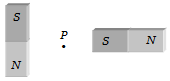1.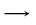2.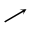3.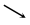4.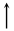Concept Questions :-

Bar magnet
High Yielding Test Series + Question Bank - NEET 2020

Difficulty Level:

A straight wire carrying a current i is turned into a circular loop. If the magnitude of the magnetic moment associated with it in M.K.S. unit is M, the length of wire will be

1. $4\mathrm{\pi iM}$                          2. $\sqrt{\frac{4\mathrm{\pi M}}{i}}$

3. $\sqrt{\frac{4\mathrm{\pi i}}{M}}$                        4. $\frac{M\mathrm{\pi }}{4i}$

Concept Questions :-

Magnetic moment
High Yielding Test Series + Question Bank - NEET 2020

Difficulty Level:

Two similar bar magnets P and Q, each of magnetic moment M, are taken. If P is cut along its axial line and Q is cut along its equatorial line, all the four pieces obtained have

1. Equal pole strength          2. Magnetic moment M/4

3. Magnetic moment M/2      4. Magnetic moment M

Concept Questions :-

Bar magnet
High Yielding Test Series + Question Bank - NEET 2020

Difficulty Level:

A magnet of magnetic moment  is placed along the x-axis in a magnetic field $\stackrel{\to }{B}=\left(0.5\stackrel{^}{i}+3.0\stackrel{^}{j}\right)T$. The torque acting on the magnet is

1. 175 $\stackrel{^}{k}$ N-m

2. 150 $\stackrel{^}{k}$ N-m

3. 75 $\stackrel{^}{k}$ N-m

4. 25$\sqrt{37}$ $\stackrel{^}{k}$ N-m

Concept Questions :-

Analogy between electrostatics and magnetostatics
High Yielding Test Series + Question Bank - NEET 2020

Difficulty Level:

Two lines of force due to a bar magnet

1. Intersect at the neutral point

2. Intersect near the poles of the magnet

3. Intersect on the equatorial axis of the magnet

4. Do not intersect at all

Concept Questions :-

Magnetic field and field lines
High Yielding Test Series + Question Bank - NEET 2020

Difficulty Level:

The magnetic field lines due to a bar magnet are correctly shown in

1.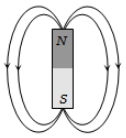2.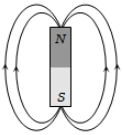3.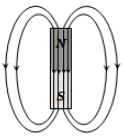4.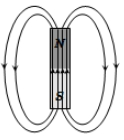Concept Questions :-

Magnetic field and field lines